# Programming help ---- python

#### IT kolla

##### Active member
• Guys මේ මගේ moratuwa uni එකෙන් free කරන පයිතන් කෝස් එකේ assignment part 1 එක. මෙකේ තියෙන්නෙ සරල කැල්කියුලේටර් එකක් හදන්න. මම මේක කෝඩ් කලා. මම මැනුවල් python software එකෙන් කරද්දි ඒ වැඩේ වෙන්වා. ඒත් එයලගේ editor එකේ දැම්මම වැරදි කියනවා. මේකට හේතුව පොඩ්ඩ්ක් බලල කියන්නකො මට. තව අන්තිම එකේ EOFError කියලා එකක් එනවා. ඒකත් ගූගල් කලාට හොයාගන්න බැරි උනා. පොඩි උපදෙස් ටිකක්
දෙන්නකෝ මේක හදාගන්න.

Course Level Programming Assignment - Programming a Calculator using Python

In this assignment you will write a computer program from scratch using the Python programming language. This program will function as a simple calculator.

Objectives

• Write a simple Python program that performs arithmetic operations based on the user input
Stage 1: A simple calculator

Your calculator should provide the following arithmetic and control operations.
• Arithmetic Operations
• Subtraction (-) subtract(a,b)
• Multiplication (*) multiply(a,b)
• Division (/) divide(a,b)
• Power (^) power(a,b)
• Remainder (%) remainder(a,b)
• Control Operations
• Terminate (#)
• Reset (\$)
Write a function select_op(choice) to select the appropriate mathematics function based on the users selection.
The behavior of the program should be as follows:
• Once the user inputs/selects an arithmetic operation, the program should ask the user to enter the two operands one by one, separated by Enter key. If the user made a mistake while entering the parameters, he can return to main menu by pressing ‘\$’ key at the end of the input string, followed by the Enter key
• Calculate the result and display the result. Inputs need to be processed as floating point values, even thought the values entered are integers. Example: 2.0 + 4.0 = 6.0
• All possible errors (at input or at generation of result) should be handled by the program
• Examples:
• Anything other than a number as operand input
• Anything other than +, -, *, /, ^ and % as arithmetic operators
• Anything other than # and \$ as control operators
• Division by zero
• The program should keep running until it is stopped by the user (using the terminate command #)
• Section 1:
• Input Arithmetic operation
• Reset or Termination
• Section 2:
• Input first operand
• Input second operand
• Reset or Termination
Task 2: Implement functions that performs given arithmetic operation on the given operands and produces the result
• Arithmetic operation and the two operands as parameters
• Return the result of the arithmetic operation
Task 3: Call the calculation function by passing user input to select_op(choice) and display the result from within the select_op(choice) function
Here are some of the messages you might want to display to the users at certain occasions. Copy and paste them as necessary in your code in appropriate situations to help with auto-grading. If there is any differences between the output of your code and the expected output, it will be displayed once you click the "Check" button. You can click on "Show differences" button to highlight the difference between outputs. This will be helpful for you to change your code to match the expected output.

"Enter first number: "
"Enter second number: "
"Not a valid number,please enter again"
"Unrecognized operation"
"Something Went Wrong"

.....................my coding...........................

while True:
print("Select operation.")
print("2.Subtract : - ")
print("3.Multiply : * ")
print("4.Divide : / ")
print("5.Power : ^ ")
print("6.Remainder: % ")
print("7.Terminate: # ")
print("8.Reset : \$ ")

return x+y

def substract(x,y):
return x-y

def multiply(x,y):
return x*y

def divide(x,y):
return x/y

def power(x,y):
return x**y

def remainder(x,y):
return x%y

# take input from the user
choice = input("Enter choice(+,-,*,/,^,%,#,\$): ")
if choice == "#":
#program ends here
print("\nDone. Terminating")
exit()
if choice == "\$":
continue
try:
a=float(input("\nEnter first number: "))
b=float(input("\nEnter second number: \n"))
except:
continue

if choice == "+":

elif choice == "-":
print(a, "-", b, "=", substract(a,b))

elif choice == "*":
print(a, "*", b, "=", multiply(a,b))

elif choice == "/":
if b == 0.0:
print("float division by zero")
else:
print(a, "/", b, "=", divide(a,b))

elif choice == '^':
print(a, "^", b, "=", power(a,b))

elif choice == "%":
print(a, "%", b, "=", remainder(a,b))

else :
print("Unrecognized operation")

.....................my coding...........................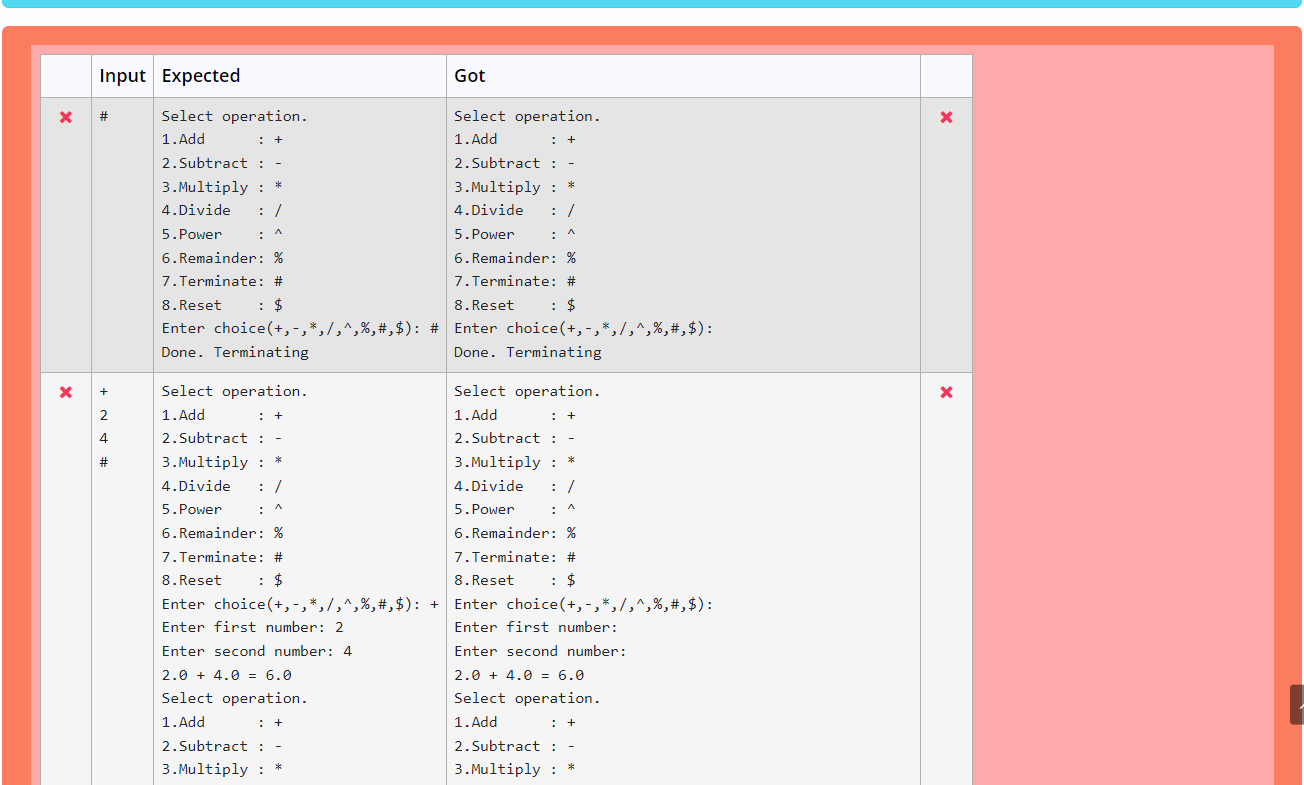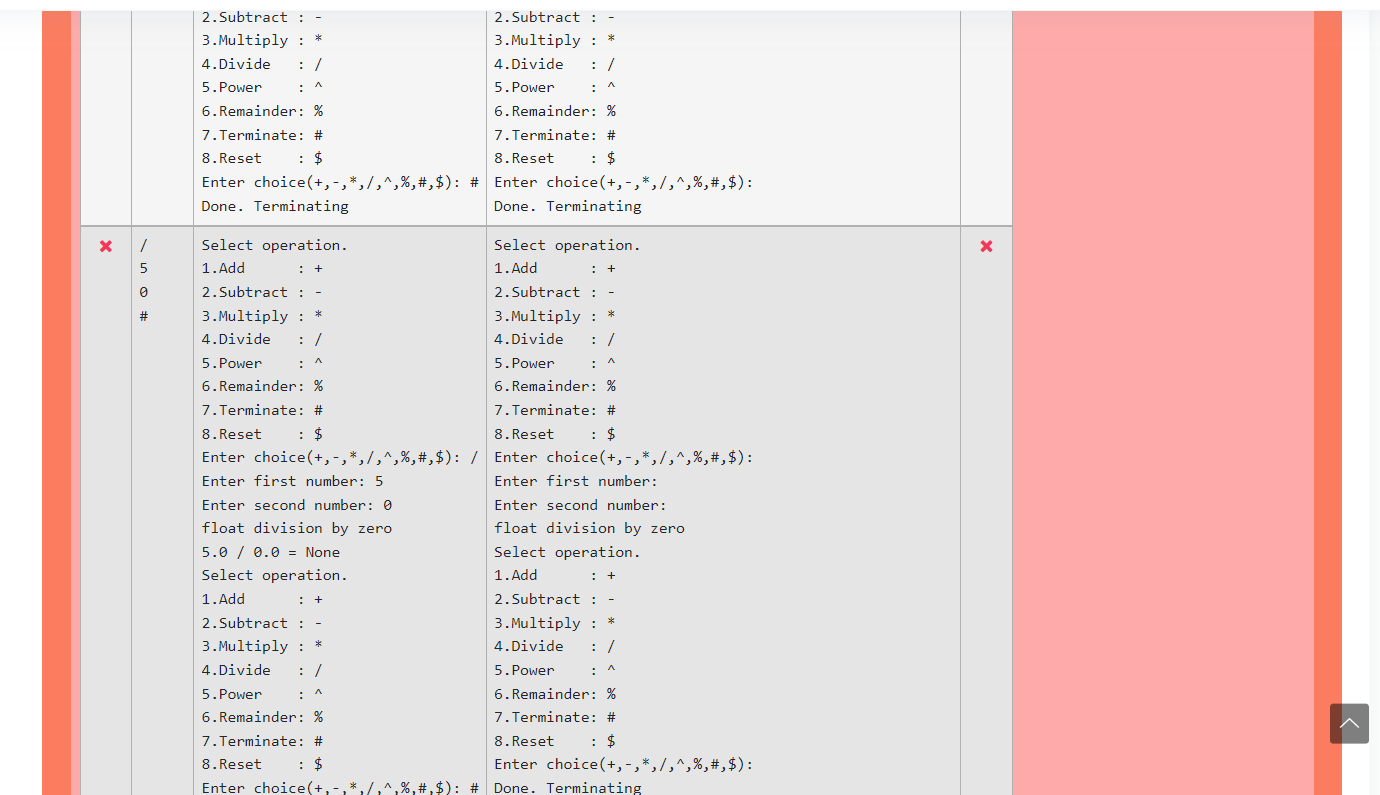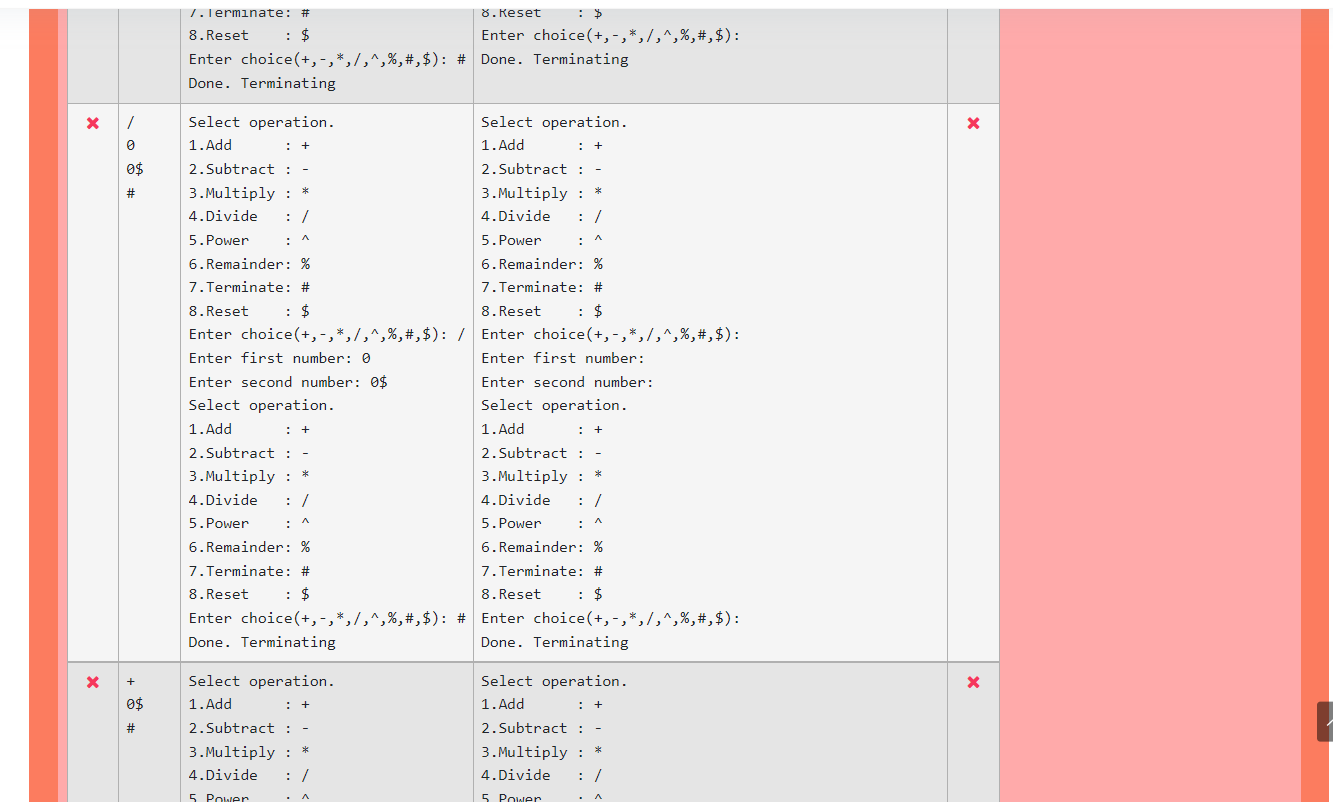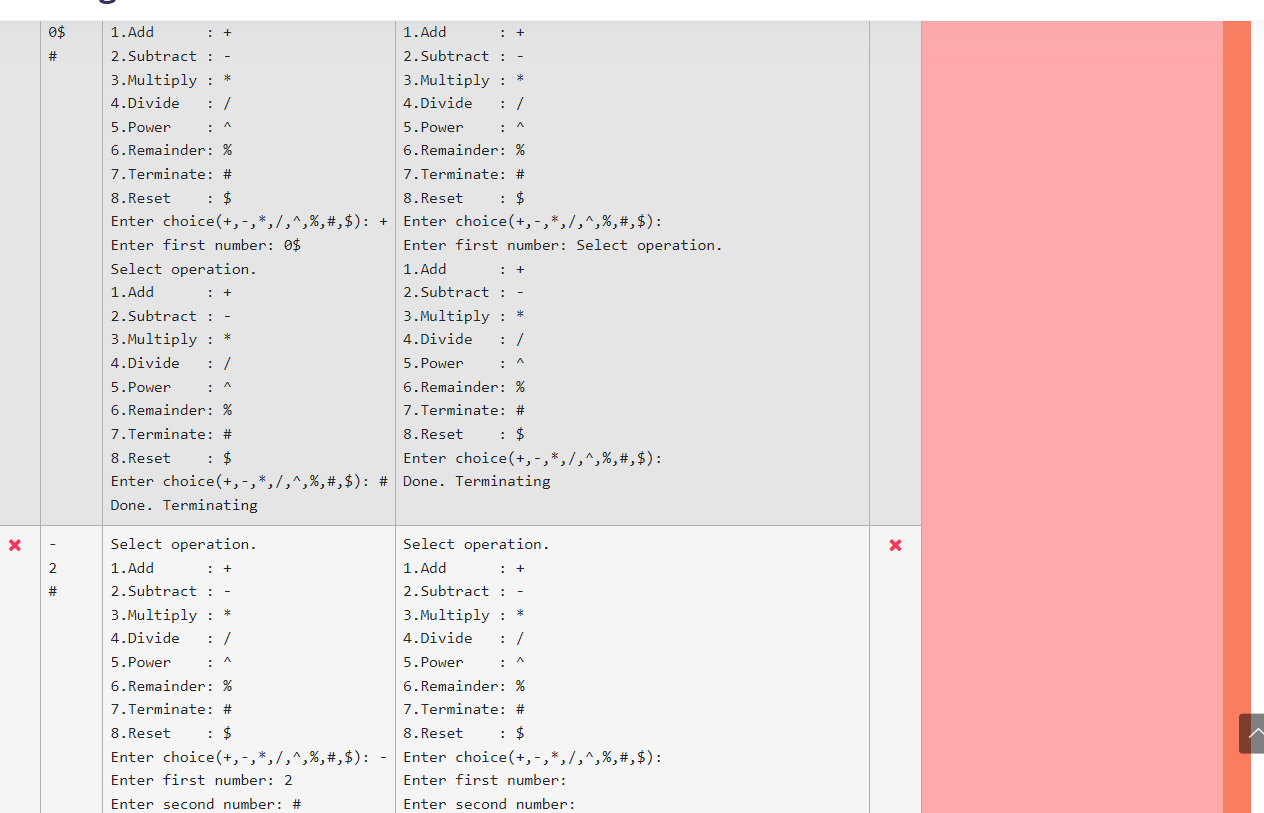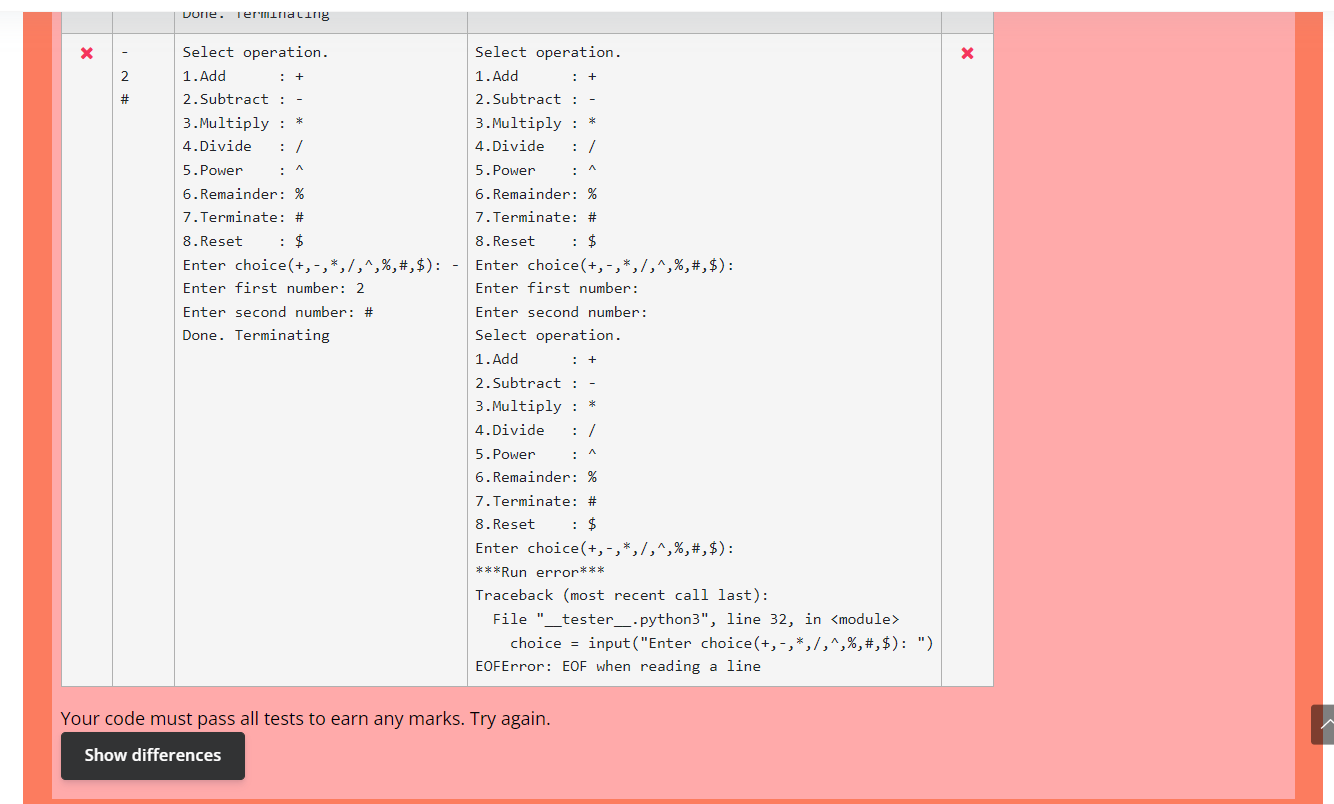•jennt

• #### IT kolla

##### Active member

• Handle this,

add try-catch, print this under catch
මට try catch ,එක දාන තැනයි හිතාගන්න බැරි. මට හිතෙන්නේ EOFError එකත් එන්නෙ වැරදි තැනින් try except දාපු නිසා වෙන්න ඇති කියල හිතෙනවා.
------ Post added on May 7, 2022 at 1:57 AM

•siri_ayya

#### BinDT

##### Well-known member
• Guys මේ මගේ moratuwa uni එකෙන් free කරන පයිතන් කෝස් එකේ assignment part 1 එක. මෙකේ තියෙන්නෙ සරල කැල්කියුලේටර් එකක් හදන්න. මම මේක කෝඩ් කලා. මම මැනුවල් python software එකෙන් කරද්දි ඒ වැඩේ වෙන්වා. ඒත් එයලගේ editor එකේ දැම්මම වැරදි කියනවා. මේකට හේතුව පොඩ්ඩ්ක් බලල කියන්නකො මට. තව අන්තිම එකේ EOFError කියලා එකක් එනවා. ඒකත් ගූගල් කලාට හොයාගන්න බැරි උනා. පොඩි උපදෙස් ටිකක්
දෙන්නකෝ මේක හදාගන්න.

Course Level Programming Assignment - Programming a Calculator using Python

In this assignment you will write a computer program from scratch using the Python programming language. This program will function as a simple calculator.

Objectives

• Write a simple Python program that performs arithmetic operations based on the user input
Stage 1: A simple calculator

Your calculator should provide the following arithmetic and control operations.
• Arithmetic Operations
• Subtraction (-) subtract(a,b)
• Multiplication (*) multiply(a,b)
• Division (/) divide(a,b)
• Power (^) power(a,b)
• Remainder (%) remainder(a,b)
• Control Operations
• Terminate (#)
• Reset (\$)
Write a function select_op(choice) to select the appropriate mathematics function based on the users selection.
The behavior of the program should be as follows:
• Once the user inputs/selects an arithmetic operation, the program should ask the user to enter the two operands one by one, separated by Enter key. If the user made a mistake while entering the parameters, he can return to main menu by pressing ‘\$’ key at the end of the input string, followed by the Enter key
• Calculate the result and display the result. Inputs need to be processed as floating point values, even thought the values entered are integers. Example: 2.0 + 4.0 = 6.0
• All possible errors (at input or at generation of result) should be handled by the program
• Examples:
• Anything other than a number as operand input
• Anything other than +, -, *, /, ^ and % as arithmetic operators
• Anything other than # and \$ as control operators
• Division by zero
• The program should keep running until it is stopped by the user (using the terminate command #)
• Section 1:
• Input Arithmetic operation
• Reset or Termination
• Section 2:
• Input first operand
• Input second operand
• Reset or Termination
Task 2: Implement functions that performs given arithmetic operation on the given operands and produces the result
• Arithmetic operation and the two operands as parameters
• Return the result of the arithmetic operation
Task 3: Call the calculation function by passing user input to select_op(choice) and display the result from within the select_op(choice) function
Here are some of the messages you might want to display to the users at certain occasions. Copy and paste them as necessary in your code in appropriate situations to help with auto-grading. If there is any differences between the output of your code and the expected output, it will be displayed once you click the "Check" button. You can click on "Show differences" button to highlight the difference between outputs. This will be helpful for you to change your code to match the expected output.

"Enter first number: "
"Enter second number: "
"Not a valid number,please enter again"
"Unrecognized operation"
"Something Went Wrong"

.....................my coding...........................

while True:
print("Select operation.")
print("2.Subtract : - ")
print("3.Multiply : * ")
print("4.Divide : / ")
print("5.Power : ^ ")
print("6.Remainder: % ")
print("7.Terminate: # ")
print("8.Reset : \$ ")

return x+y

def substract(x,y):
return x-y

def multiply(x,y):
return x*y

def divide(x,y):
return x/y

def power(x,y):
return x**y

def remainder(x,y):
return x%y

# take input from the user
choice = input("Enter choice(+,-,*,/,^,%,#,\$): ")
if choice == "#":
#program ends here
print("\nDone. Terminating")
exit()
if choice == "\$":
continue
try:
a=float(input("\nEnter first number: "))
b=float(input("\nEnter second number: \n"))
except:
continue

if choice == "+":

elif choice == "-":
print(a, "-", b, "=", substract(a,b))

elif choice == "*":
print(a, "*", b, "=", multiply(a,b))

elif choice == "/":
if b == 0.0:
print("float division by zero")
else:
print(a, "/", b, "=", divide(a,b))

elif choice == '^':
print(a, "^", b, "=", power(a,b))

elif choice == "%":
print(a, "%", b, "=", remainder(a,b))

else :
print("Unrecognized operation")

.....................my coding...........................

View attachment 170037
View attachment 170038
View attachment 170039
View attachment 170040
View attachment 170041
Oke usergen ganna input aimath print wenna ona bn.kiriyema thread ekak thibba meeka gana poddak balapan.

•siri_ayya and shenat

#### shenat

##### Well-known member
• Oke usergen ganna input aimath print wenna ona bn.kiriyema thread ekak thibba meeka gana poddak balapan.

Ow menna meka mehema wenna ona. Nattan weradi kiyanawa

#### pasindu201

##### Well-known member
• hodata balanna expected uotput ekai,oyage output ekei thiyena wenasa gana,a dekama hariyatama samana wenna one naththam pass wenne na,hodata baluwoth oyata penei expected output eke user inout karana options eka penwa,oyage output eke ehema penne na,eka hadalla ayemath submit karala balanna,

#### AslalShaja

##### Member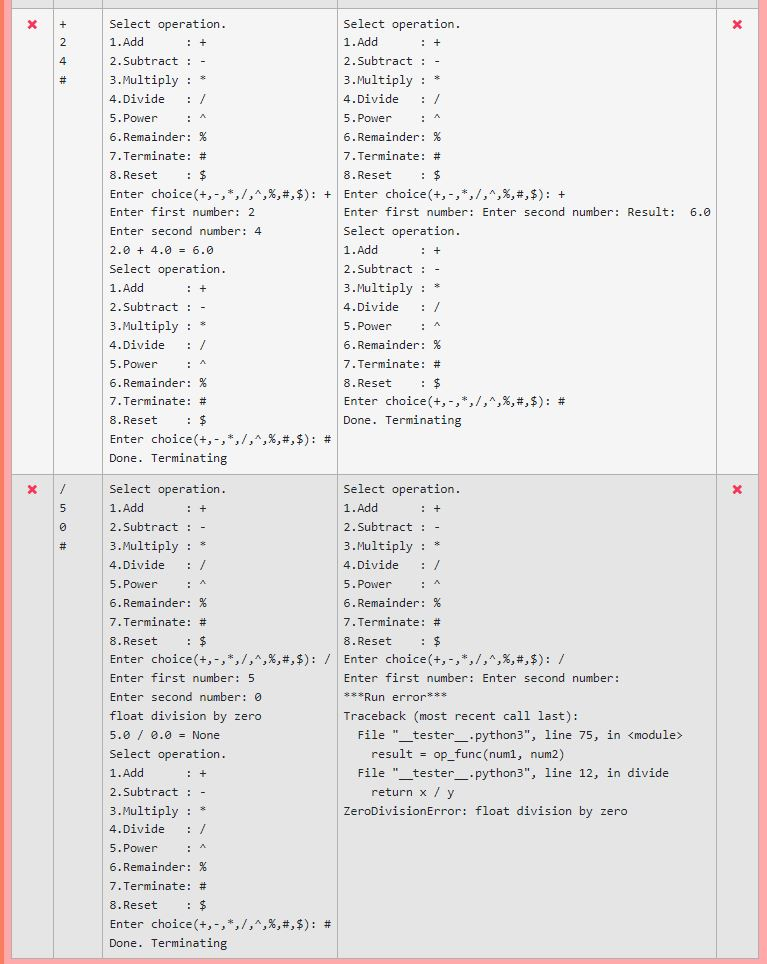I got these errors